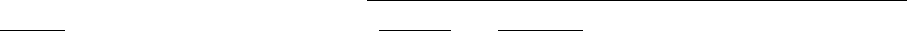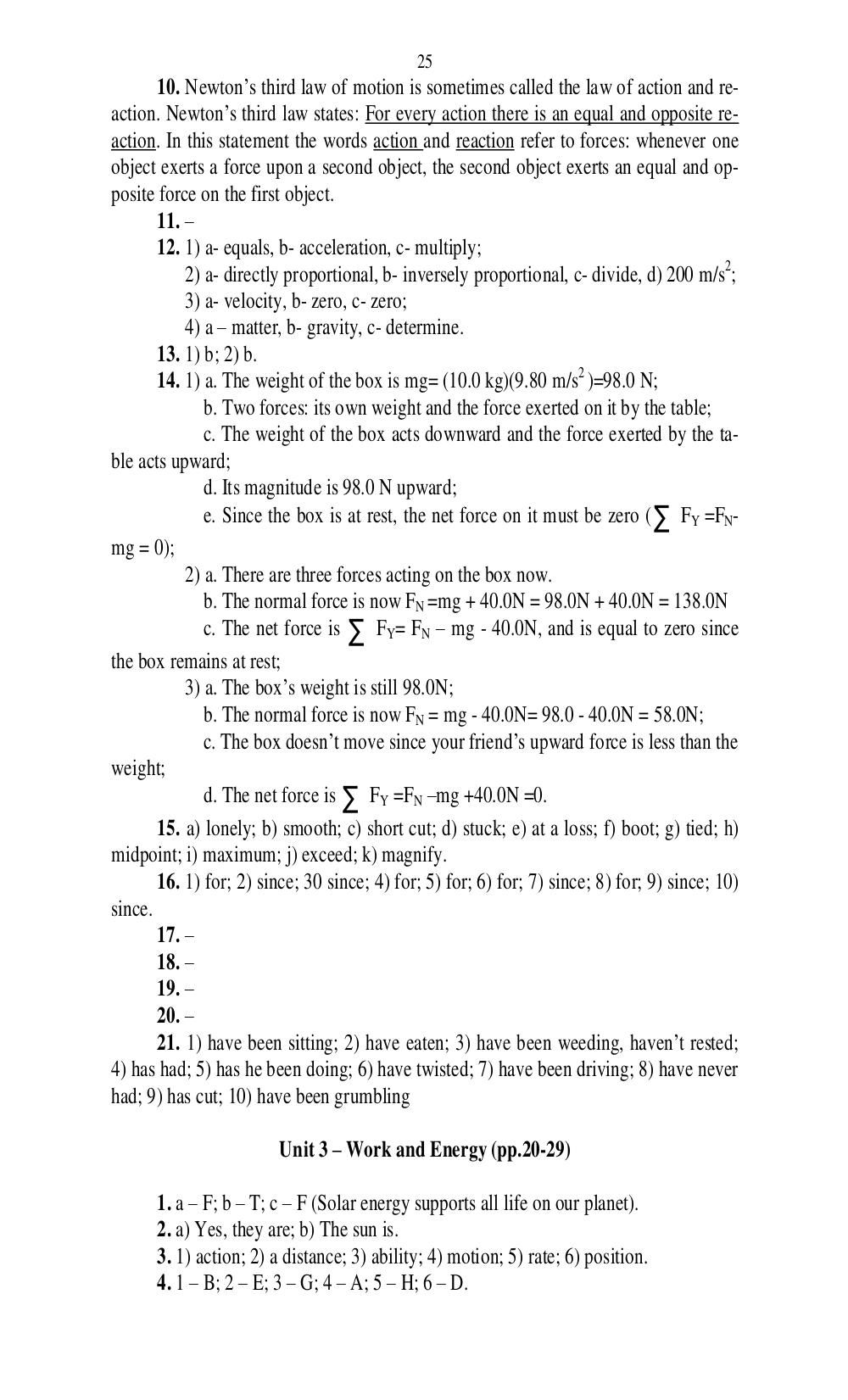# Английский язык. Ч. 1. Ильичева Н.А - 25 стр.

Составители:

Рубрика:

• ## Иностранный язык25
10. Newtons third law of motion is sometimes called the law of action and re-
action. Newtons third law states: For every action there is an equal and opposite re-
action. In this statement the words action and reaction refer to forces: whenever one
object exerts a force upon a second object, the second object exerts an equal and op-
posite force on the first object.
11.
12. 1) a- equals, b- acceleration, c- multiply;
2) a- directly proportional, b- inversely proportional, c- divide, d) 200 m/s
2
;
3) a- velocity, b- zero, c- zero;
4) a matter, b- gravity, c- determine.
13. 1) b; 2) b.
14. 1) a. The weight of the box is mg= (10.0 kg)(9.80 m/s
2
)=98.0 N;
b. Two forces: its own weight and the force exerted on it by the table;
c. The weight of the box acts downward and the force exerted by the ta-
ble acts upward;
d. Its magnitude is 98.0 N upward;
e. Since the box is at rest, the net force on it must be zero (
F
Y
=F
N
-
mg = 0);
2) a. There are three forces acting on the box now.
b. The normal force is now F
N
=mg + 40.0N = 98.0N + 40.0N = 138.0N
c. The net force is
F
Y
= F
N
mg - 40.0N, and is equal to zero since
the box remains at rest;
3) a. The boxs weight is still 98.0N;
b. The normal force is now F
N
= mg - 40.0N= 98.0 - 40.0N = 58.0N;
c. The box doesnt move since your friends upward force is less than the
weight;
d. The net force is
F
Y
=F
N
mg +40.0N =0.
15. a) lonely; b) smooth; c) short cut; d) stuck; e) at a loss; f) boot; g) tied; h)
midpoint; i) maximum; j) exceed; k) magnify.
16. 1) for; 2) since; 30 since; 4) for; 5) for; 6) for; 7) since; 8) for; 9) since; 10)
since.
17.
18.
19.
20.
21. 1) have been sitting; 2) have eaten; 3) have been weeding, havent rested;
4) has had; 5) has he been doing; 6) have twisted; 7) have been driving; 8) have never
had; 9) has cut; 10) have been grumbling
Unit 3 Work and Energy (pp.20-29)
1. a F; b T; c F (Solar energy supports all life on our planet).
2. a) Yes, they are; b) The sun is.
3. 1) action; 2) a distance; 3) ability; 4) motion; 5) rate; 6) position.
4. 1 B; 2 E; 3 G; 4 A; 5 H; 6 D.25
10. Newton’s third law of motion is sometimes called the law of action and re-
action. Newton’s third law states: For every action there is an equal and opposite re-
action. In this statement the words action and reaction refer to forces: whenever one
object exerts a force upon a second object, the second object exerts an equal and op-
posite force on the first object.
11. –
12. 1) a- equals, b- acceleration, c- multiply;
2) a- directly proportional, b- inversely proportional, c- divide, d) 200 m/s2;
3) a- velocity, b- zero, c- zero;
4) a – matter, b- gravity, c- determine.
13. 1) b; 2) b.
14. 1) a. The weight of the box is mg= (10.0 kg)(9.80 m/s2 )=98.0 N;
b. Two forces: its own weight and the force exerted on it by the table;
c. The weight of the box acts downward and the force exerted by the ta-
ble acts upward;
d. Its magnitude is 98.0 N upward;
e. Since the box is at rest, the net force on it must be zero ( ∑ FY =FN-
mg = 0);
2) a. There are three forces acting on the box now.
b. The normal force is now FN =mg + 40.0N = 98.0N + 40.0N = 138.0N
c. The net force is ∑ FY= FN – mg - 40.0N, and is equal to zero since
the box remains at rest;
3) a. The box’s weight is still 98.0N;
b. The normal force is now FN = mg - 40.0N= 98.0 - 40.0N = 58.0N;
c. The box doesn’t move since your friend’s upward force is less than the
weight;
d. The net force is ∑ FY =FN –mg +40.0N =0.
15. a) lonely; b) smooth; c) short cut; d) stuck; e) at a loss; f) boot; g) tied; h)
midpoint; i) maximum; j) exceed; k) magnify.
16. 1) for; 2) since; 30 since; 4) for; 5) for; 6) for; 7) since; 8) for; 9) since; 10)
since.
17. –
18. –
19. –
20. –
21. 1) have been sitting; 2) have eaten; 3) have been weeding, haven’t rested;
4) has had; 5) has he been doing; 6) have twisted; 7) have been driving; 8) have never
had; 9) has cut; 10) have been grumbling

Unit 3 – Work and Energy (pp.20-29)

1. a – F; b – T; c – F (Solar energy supports all life on our planet).
2. a) Yes, they are; b) The sun is.
3. 1) action; 2) a distance; 3) ability; 4) motion; 5) rate; 6) position.
4. 1 – B; 2 – E; 3 – G; 4 – A; 5 – H; 6 – D.